Courses
Courses for Kids
Free study material
Free LIVE classes
More

# JEE Advanced 2023 Revision Notes for Alcohols, Phenols and EthersLIVE
Join Vedantu’s FREE Mastercalss

Alcohols, Phenols, and Ethers are among the fundamental compounds studied in organic chemistry. This chapter is very important for the JEE Advanced exam. In fact, these compounds are taught to develop a conceptual understanding of organic chemistry among students. They will learn the physical and chemical properties of these compounds and how they behave in different chemical conditions. Download and refer to Alcohols Phenols Ethers JEE Advanced material PDF for free from Vedantu and prepare for your exam.

These revision notes have been prepared by the subject experts at Vedantu to offer a simple explanation of all concepts and fundamental principles of this chapter. Students will learn how and why these compounds behave in different organic reactions and what the products are.

Last updated date: 22nd Sep 2023
Total views: 138.9k
Views today: 4.38k

## Access JEE Advanced 2023 Revision Notes Chemistry Alcohols, Phenols and Ethers

Alcohols are the hydroxyl derivatives of hydrocarbons, i.e., obtained by the replacement of one or more H atoms ${\text{R - H}}\xrightarrow[{{\text{ + OH}}}]{{{\text{ - H}}}}{\text{R - OH}}$.

• The organic compounds in which a hydroxyl group is attached to a carbon atom are called alcohols.

• The compound in which the hydroxyl group is attached to an unsaturated carbon atom of a double bond is called enols.

### Classification:

Alcohol is classified according to the number of hydroxyl groups in its molecules.

• Monohydric: alcohols contain one-OH group, dihydric two-OH group and trihydric three-OH group.

• Monohydric: $R - C{H_2}OH$

• Dihydric alcohol: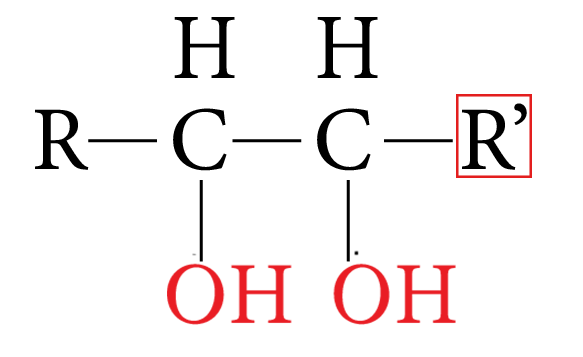Dihydric Alcohol

Monohydric alcohols are further classified according to the hybridization of the carbon atom to which the -OH group is attached.

Compounds containing ${C_{s{p^3}}} - OH$ bond:

In these alcohols, the -OH group is attached to $s{p^3}$ hybridised carbon atom of the alkyl group.

These are further subdivided as follows:

### 1. Primary Alcohol (1°):

• In these alcohols the OH group is attached to the primary carbon atom.

• They posses a general formula $RC{H_2}OH$. R may be H in the first member and alkyl group in the rest of the members

Example:

$HC{H_2}OH$ (Methyl alcohol)

$C{H_3}C{H_2}OH$ (Ethyl alcohol)

$C{H_3}C{H_2}C{H_2}OH$ (n-Propyl alcohol)

### 2. Secondary Alcohol (2°)

• In these alcohols the OH group is attached with a secondary carbon atom. They posses a general form $R_2CHOH$

• Isopropyl alcohol secondary butyl alcohol

Example: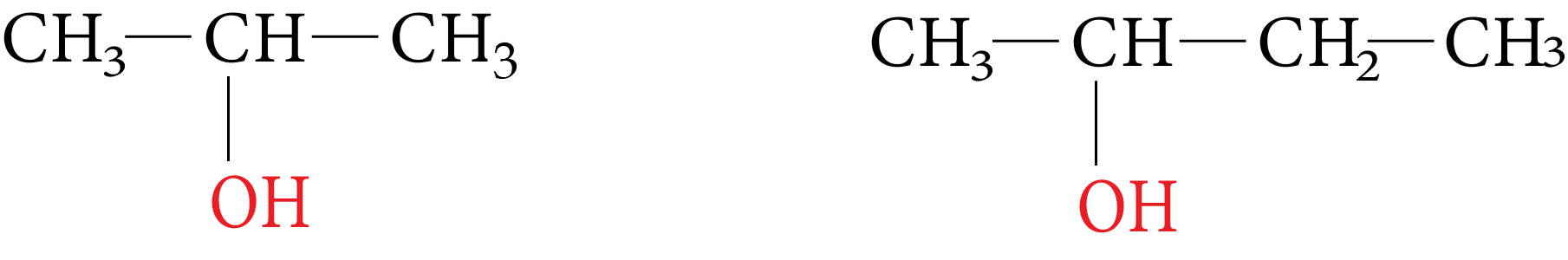Iso Propyl and Secondary Butyl Alcohol

### 3. Tertiary Alcohol (3°)

• In these alcohols the -OH group is attached with tertiary carbon atom they posses a general formula ${R_3}C - OH$

Example:Tertiary Alcohol

### Isomerism:

Alcohols may exhibit chain, position, and functional isomerism.

Functional isomers of alcohols are ethers.

Examples: $C{H_3} - C{H_2} - OH$ and $C{H_3} - O - C{H_3}$ are functional isomers.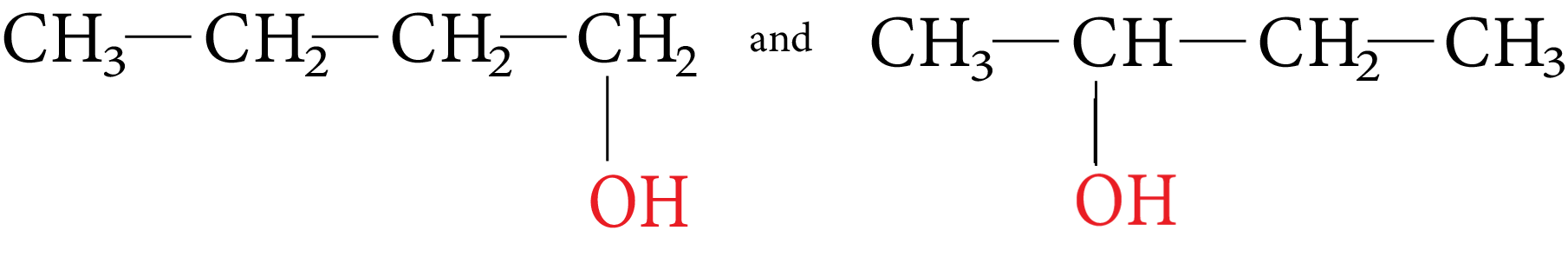Position Isomers

• Unsaturated alcohols and carbonyl compounds are tautomers.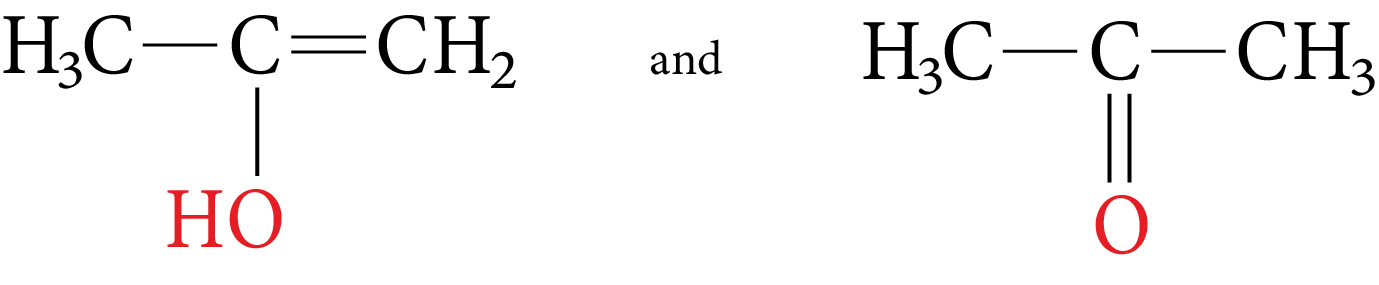Tautomers

• Alcohols containing chiral centres can exhibit optical isomerism.

• The simplest monohydric alcohol that can exhibit enantiomeric is butan-2-ol.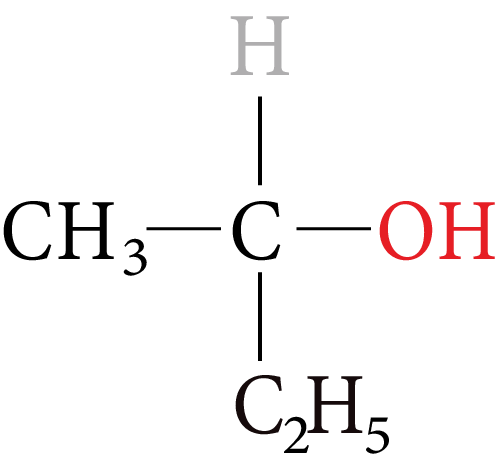Monohydric Alcohol

• Gauche form of glycol is the most stable. This is due to intramolecular hydrogen bonding

### Physical Properties of Alcohols:

• Alcohols have higher boiling points than hydrocarbon, ether, aldehydes and ketones of comparable molecular masses.

• Alcohols have greater intermolecular attraction arising due to intermolecular hydrogen bonds. The tendency of formation of hydrogen bonds.

• The tendency of formation of hydrogen bond is 1o  > 2o  > 3o alcohols.

• The boiling points of alcohol increase with an increase in molecular mass arising due to Vander Waals force.

• Alcohols and ethers are functional isomers but the boiling point of alcohol is greater than the corresponding ether due to the formation of intermolecular hydrogen bonds.

Example: Methanal<Ethanol<Propanol<Butanol.

• In the case of alcohol with the same alkyl group, the boiling point decreases with increasing branching.

Example: n-Butyl alcohol>Iso butyl alcohol>tertbutyl alcohol.

• Alcohols are soluble in water due to hydrogen bonding between molecules of alcohol and water.

• Solubility in water decreases with an increase in the size of the non-polar hydrophobic alkyl group, which resists dissolution.

• Among isomeric alcohol, solubility decreases with an increase in surface area

Example: tertbutyl alcohol>Isobutyl alcohol>n-Butyl alcohol

### Chemical Properties:

• The hydroxyl group present in alcohols is a very reactive group and the characteristic reactions of alcohols are the reaction of -OH group.

• The reaction of the hydroxyl group consists of either cleavage of the C-O bond or the cleavage of the O-H bond.

• C-O bond is weaker in the case of tertiary alcohol due to the +I effect of the alkyl group.

• Hence in O-H cleavage, the order of the reaction is primary>secondary>Tertiary and in C-O cleavage the order of reactivity is Tertiary>secondary>primary.

• During O-H cleavage proton is given out showing that alcohols are acidic in nature.

• However, alcohols are weaker acids than water. This is because the alkyl groups in alcohols have a +I effect.

• Hence the following order is followed in the acidic nature.

CH3 >1o alcohol > 2o alcohol > 3o alcohol

### Methods of Distinguishing Between Primary, Secondary, and Tertiary Alcohols:

 Test Primary alcohol Secondary alcohol Tertiary alcohol Luca’s reagent:Testing with a mixture of conc. HCl and anhydrous $ZnC{l_2}$. Does not form turbidity at room temperature (turbidity is obtained only upon heating) Turbidity is formed in 5 to 10 minutes Turbidity is formed immediately Catalytic dehydrogenation:(Vapours of alcohol are passes over hot metal). $RC{H_2}OH\xrightarrow[{300^\circ C}]{{Cu}}RCHO$An aldehyde is obtained $RCHOHR\xrightarrow[{300^\circ C}]{{Cu}}RCOR$A ketone is obtained An alkene is obtained Victor Meyer test:${\text{Alcohol}}\xrightarrow{{{\text{HI}}}}{\text{Alkyl iodide}}$$\xrightarrow{{{\text{AgN}}{{\text{O}}_{\text{2}}}}}{\text{Nitro compounds}}$$\xrightarrow{{\left( i \right)HN{O_2}}}\xrightarrow{{\left( {ii} \right)NaOH}}$ Nitrolic acid gives red colour. Pseudo-nitro gives blue colour. No colour is obtained.

Oxidation: (Reactive C-H bonds).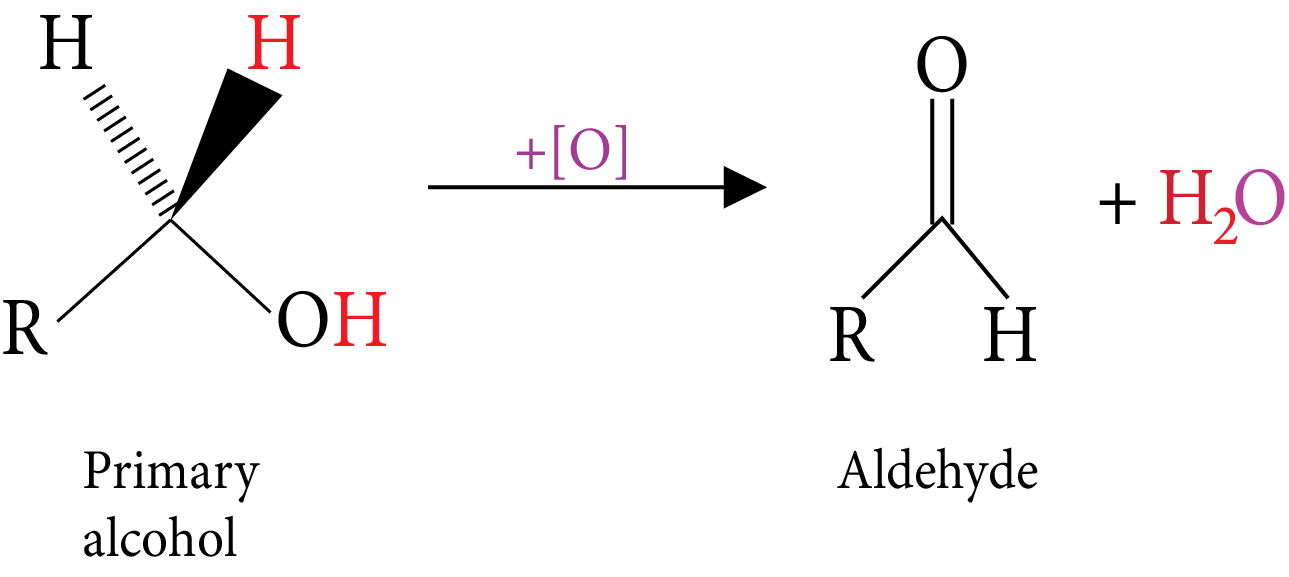Primary Alcohol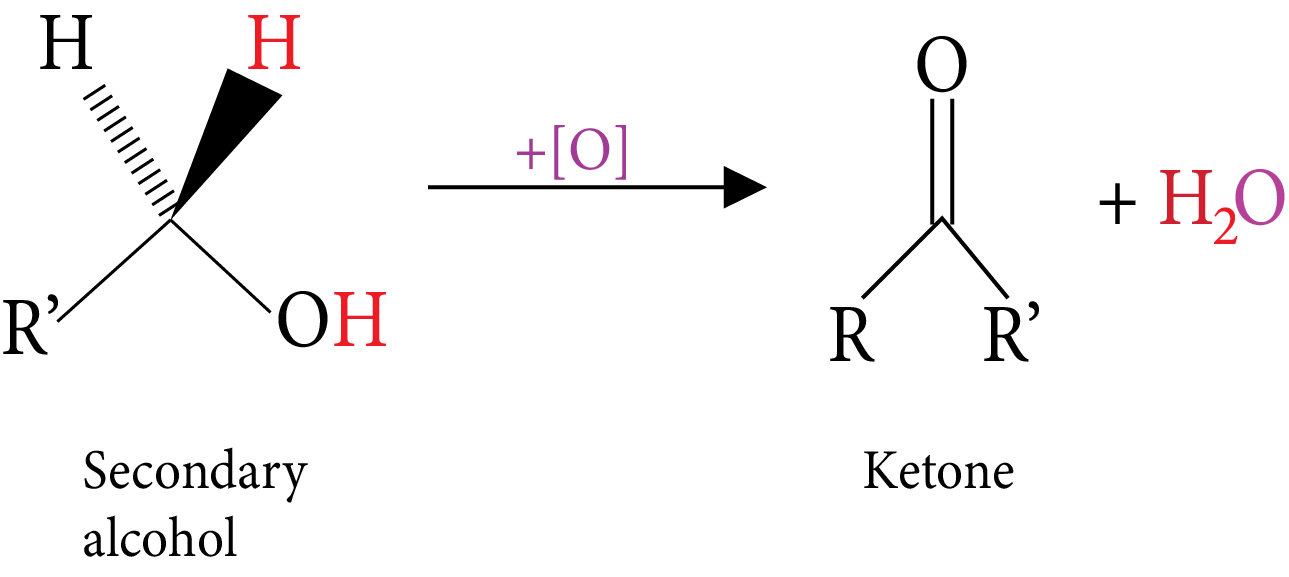Secondary Alcohol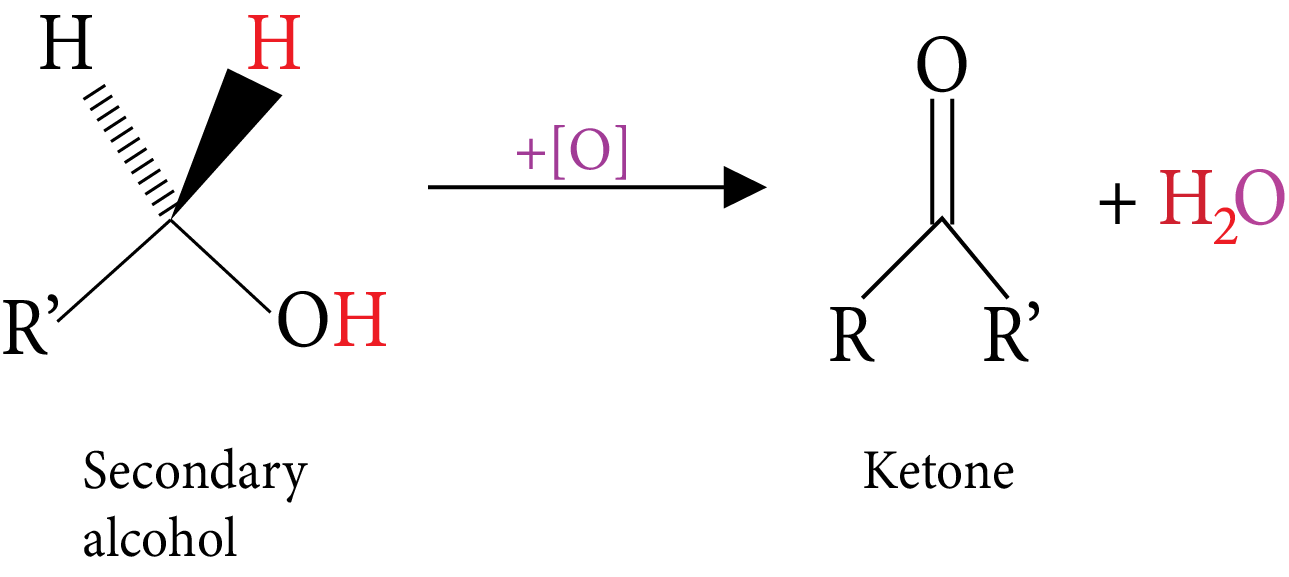Tertiary Alcohol-Oxidation

### Phenol

Aromatic hydroxy compounds in which -OH group is bonded to benzene ring directly is called Phenol. Monohydroxy benzene is called phenol. Phenol is also known as Carbolic acid.

• The simplest hydroxyl derivation of benzene is phenol which is also its accepted IUPAC name.

• Methyl phenols are called cresols.

• Dihydroxy derivatives of benzene are called benzene diols.

• The phenols, the -OH group is attached to $s{p^2}$ hybridized carbon of an aromatic ring.

• The bond angle of $C - O - H$ in Phenol is 109°.

The carbon-oxygen bond length is 136pm which is slightly less than of methanol. This is due to the partial double bond character on account of conjugation of unshared electro-pair of oxygen with the aromatic ring.

### Physical Properties:

• Phenol has a higher boiling point than the arenes or haloarenes or ethers of the same molecular weight. It is due to the formation of the intermolecular hydrogen bonds.

• Compared relative to pure aromatic hydrocarbons phenols are more soluble in water due to their ability to form hydrogen bonding with water.

• As the hydrocarbon part increases in size and mass, the solubility decreases due to the increasing hydrophobic nature.

### Chemical Properties:

• Acidic nature of phenol: Alcohols and phenols react with active metals like Na, K, Al etc to liberate hydrogen gas.

$2ROH + 2Na \to 2RONa + {H_2}$

$2{C_6}{H_5}OH + 2Na \to 2{C_6}{H_5}ONa + {H_2}$

• Phenols also react with aqueous NaOH solution to produce the salt sodium phenoxide and water.

• Relative acid strength: Carboxylic acid > Carbonic acid > phenol > Methyl alcohol > Water > Other alcohols.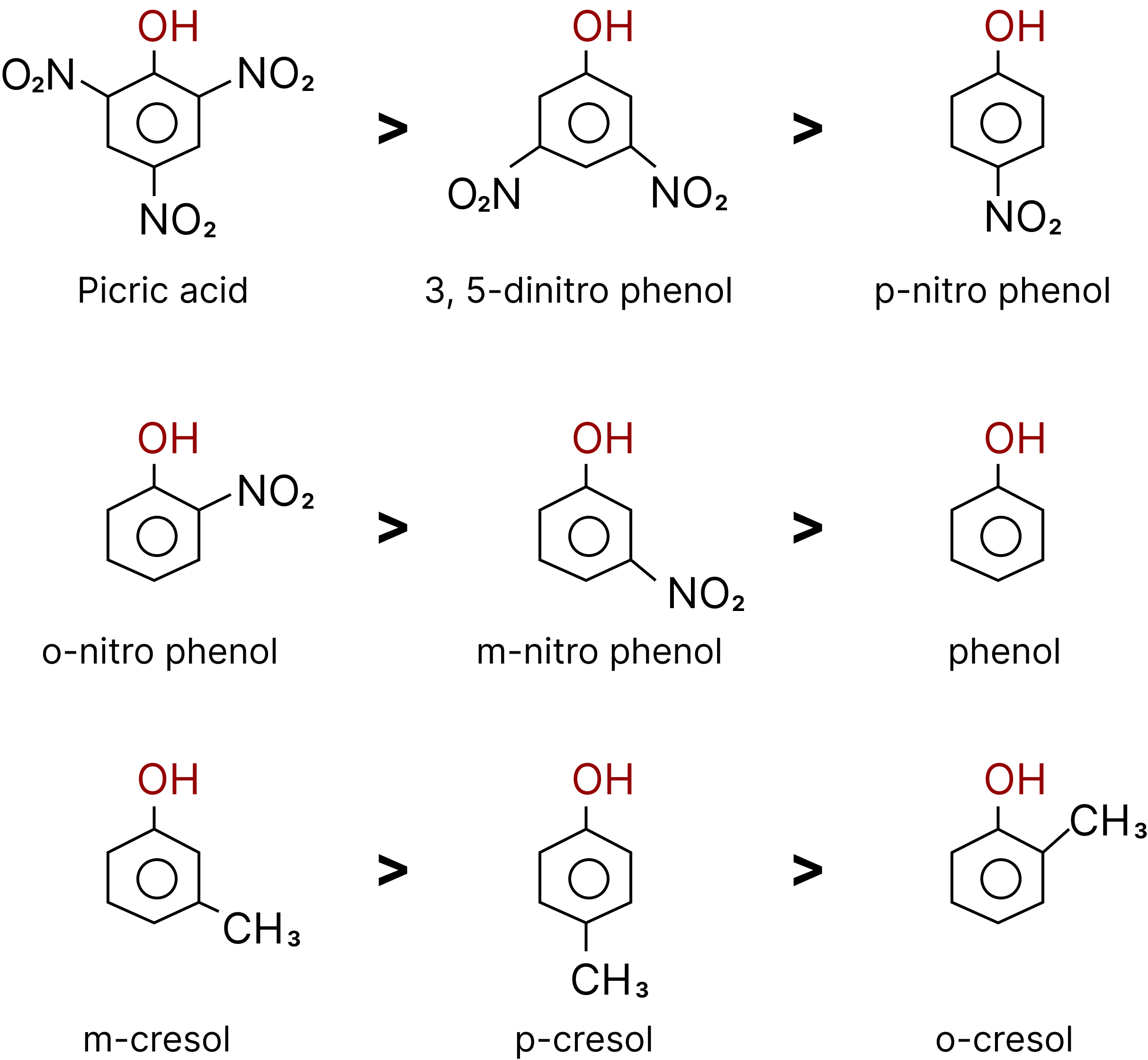Order of Acidic Strength of Phenols.

The greater the $pKa$ value, the weaker the acid.

### Esterification of Phenol:

• Phenol reacts with carboxylic acid and their derivates like acid chlorides and anhydrides to form esters. This reaction is called as Schotten-Baumann reaction.

${C_6}{H_5}OH + RCOOH\xrightarrow[{{H^ + }}]{}{C_6}{H_5} - O - CO - R + {H_2}O$

• During esterification H comes from phenol and OH comes from carboxylic acid. ${C_6}{H_5}OH + RCOCl\xrightarrow{{pyridine}}{C_6}{H_5} - O - R + HCl$.

• Salicylic acid on acetylation gives acetyl salicylic acid known as Aspirin.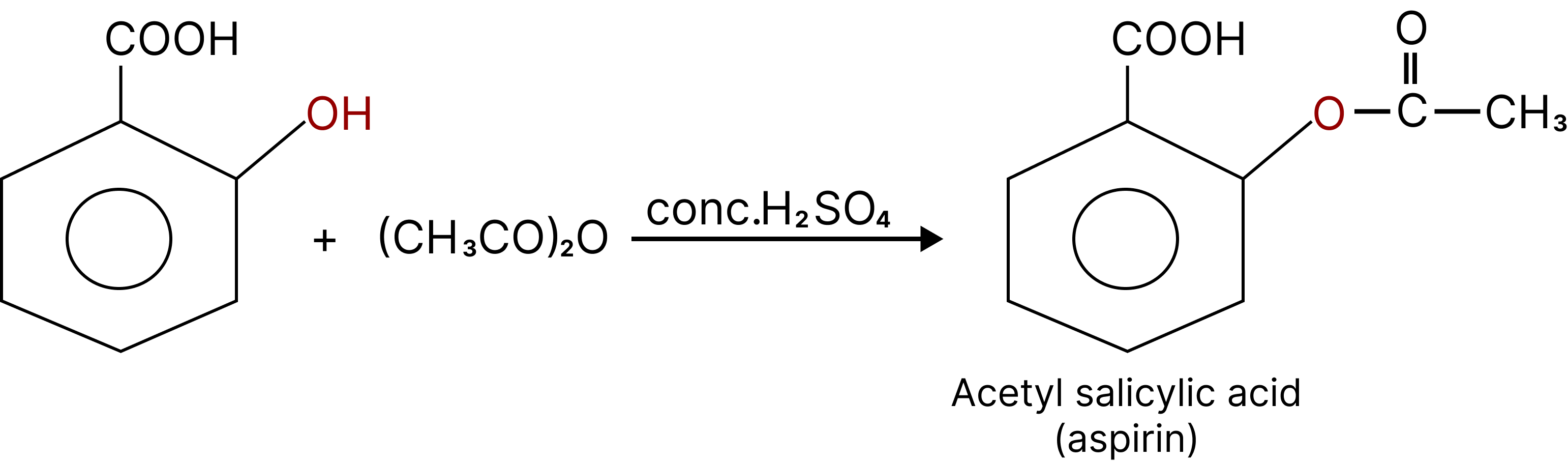Esterification of Phenol

Electrophilic aromatic substitution reaction of phenol: In phenol, -OH group is ring activating and ortho and para directing as these positions get more electron density through resonance.

### Nitration: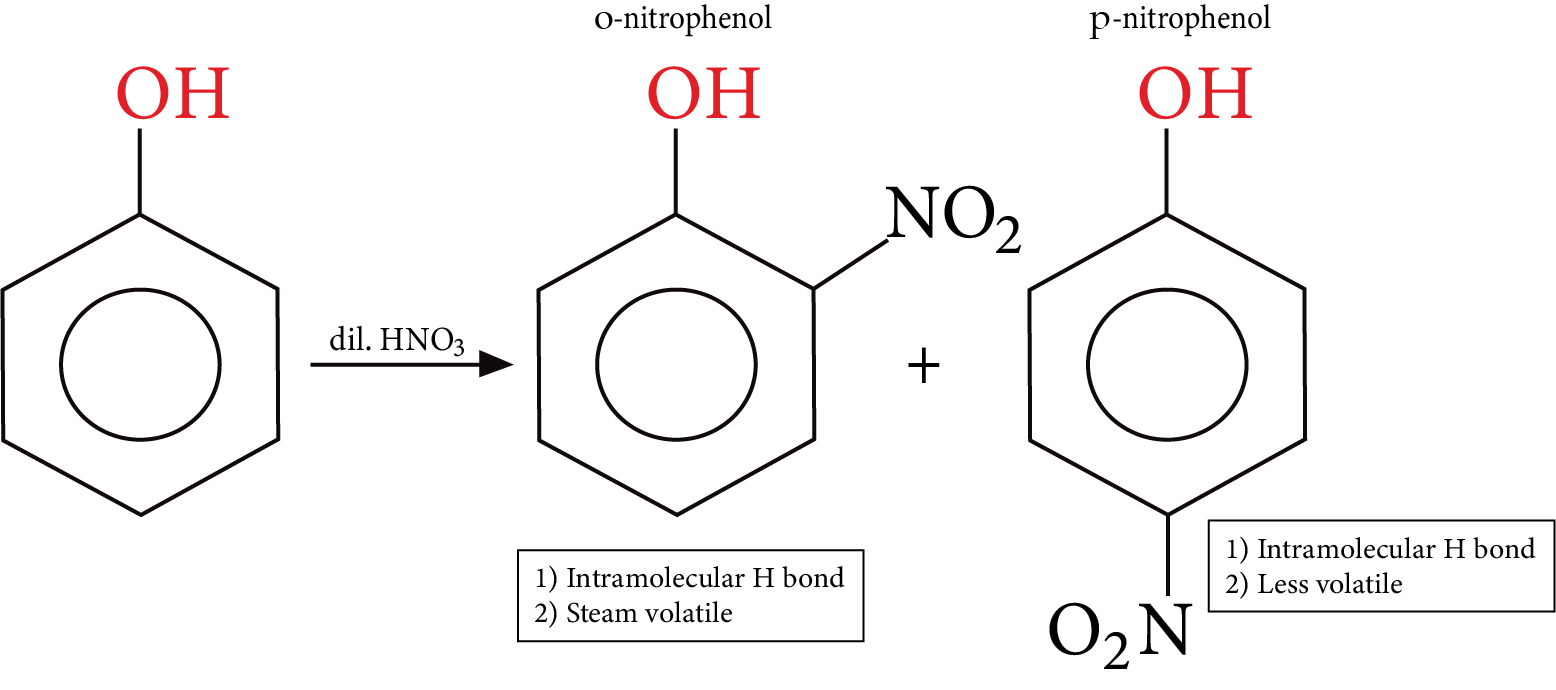Nitration of Phenol

• Phenol is a colourless solid, the colour if any associated with phenol is either due to the presence of some other functional group $- N{O_2}$ or due to the presence of some oxidized product of phenol impurity.

• Comparative solubility and boiling point are observed in the three isomers (o-m-and p) nitrophenols. p-nitrophenol is capable of forming intermolecular H bonds between themselves and with water too, Hence it has a higher melting point and more solubility in water m-nitrophenol also behaves the same way.

• Nitration of phenol gives a poor yield because nitric acid also causes oxidation of phenol, to give the unwanted product.

### Halogenation:

• When the reaction is carried out in a solvent of low polarity such as $CHC{l_3}$ or $C{S_2}$ and at low temperature, mono-bromo-phenols are formed.Halogenation of Phenol

### Uses of Phenol:

• It is the raw material for manufacturing important dyes, drugs and pharmaceuticals.

• 2,4-dichloro-3,5 dimethyl phenol is used as a powerful antiseptic under the name Dettol.

• It is used to manufacture drugs like Aspirin, Salol etc.

### Tests of Phenol:

• Phenol in water gives violet colour with a drop of $FeC{l_3}$.

• Phenol in water gives a white precipitate with bromine water.

• Phenol gives a blue colour indophenol with ammonia and sodium hypochlorite.

### Ethers

The substitution of hydrogen in a hydrocarbon by an alkoxy or aryloxy group produce ethers, General formula of ether ${C_n}{H_{2n + 2}}O$ which is similar to that of monohydric alcohols.

• Ethers are alkyl (or) Aryl derivatives of alcohol (or) Phenols, also treated as di alkyl derivatives of water.

• The general formula of ether: $R - O - {R^1}$

• R and R1 & are alkyl or aryl groups. If the R1 and R are same it is or symmetrical

Ex:- $C{H_3}O{C_2}{H_5},{\text{ }}C{H_3}O{C_3}{H_7}$

• If R and R1 are different it is called mixed or unsymmetrical ether.

Ex:- $C{H_3}OC{H_3},{\text{ }}C{H_3}O{C_2}{H_5}$

• If both R and R1 alkyl groups those are known an aliphatic ether.

Ex:- $C{H_3}OC{H_3},{\text{ }}C{H_3}O{C_2}{H_5}$

• If both R and R1 are aryl groups those are known as di aryl ethers.

Ex:- ${C_6}{H_5}O{C_6}{H_5}$

If one is alkyl other one is aryl those are known as phenolic ether.

Ex:- ${C_6}{H_5}OC{H_3}$

• Ethers are considered to be di-alkyl derivatives of water.

### Isomerism in Ethers

Functional Isomerism: Ethers are functional isomeric with a monohydric alcohol.

Example:

${{\text{C}}_{\text{2}}}{{\text{H}}_{\text{5}}}{\text{OH and C}}{{\text{H}}_{\text{3}}}{\text{OC}}{{\text{H}}_{\text{3}}}$

${{\text{C}}_3}{{\text{H}}_7}{\text{OH and C}}{{\text{H}}_{\text{3}}}{\text{O}}{{\text{C}}_2}{{\text{H}}_5}$

${{\text{C}}_6}{{\text{H}}_{\text{5}}}{\text{C}}{{\text{H}}_2}{\text{OH and }}{{\text{C}}_6}{{\text{H}}_5}{\text{OC}}{{\text{H}}_{\text{3}}}$

Metamerism: Ethers with minimum four carbons have three metamers.

Example:

1. $C{H_3}O{C_3}{H_7}$

2. ${C_2}{H_5}O{C_2}{H_5}$

3. $C{H_3}OCH{(C{H_3})_2}$

1 and 2, 2 and 3 are metamers but 1 and 3 are position isomers.

### Physical Properties:

• Ethers are polar in nature whose dipole moment$\left( \mu \right)$is not equal to zero.

• Boiling point of ethers is less than their isomeric alcohols due to the presence of hydrogen bonds in alcohols.

• Lower members of ethers are miscible in water due to hydrogen bonds with water molecules.

• Dimethyl ether, ethyl methyl ether are gases.

• Other ethers are colourless, highly volatile liquids. Diethyl ether has a characteristic pleasant odour and produces temporary unconsciousness when vapour is inhaled.

• Ethers are slightly soluble in water and readily soluble in organic solvents due to lack of H-bonding. Boiling point of Diethyl ether is less than its functional isomer butyl alcohol $\left( {{C_4}{H_9}OH} \right)$ .

• Di ethyl ether and Butanol are almost soluble water to same extent due to Hydrogen Bonding. Even both Alkyl groups are same in ether those are polar due to bent shape.

### Chemical Properties:

• Ethers are less reactive than alcohols due to the non-availability of active hydrogen.

• Ethers react with acids, phosphorous halides, oxidising agents and reducing agents at high temperatures but cannot react with metals like sodium

• Reactions of ether are classified into three types:

1. Reaction involving cleavage of C-O bond.

2. Reaction of ethereal oxygen.

3. Reaction of Epoxides.

• Ethers behave as weak lewis base due to the presence of lone pair of electrons on ethereal oxygen. therefore, dissolve in strong acids.

• Ethers are volatile and highly inflammable. They burn in air or oxygen to form carbon dioxide and water.

### Friedel Craft’s ReactionFriedel Craft’s Reaction

## Importance of Alcohols Phenols and Ethers

This chapter elaborately explains what alcohols are. The reactions that only alcohols go through to give a particular genre of products and exhibit their innate chemical properties are explained here.

You will also learn how different types of phenols are formed. This chapter covers the features the phenols have in common and how can we distinguish between them.

The ethers are an interesting class of organic compounds. In this chapter, all kinds of important reactions of Alcohol, Phenol and Ether for JEE Advanced are explained elaborately with balanced chemical equations.

This chapter is of utmost importance for JEE Advanced exam preparation as the mechanism of the reactions to prepare alcohols, phenols, and ethers are covered here. Students will learn how these compounds are prepared from the given reactants. They will realize and imbibe the concept of organic chemistry better with the knowledge of this chapter.

They will learn the physical and chemical properties of Alcohols, Phenols, and Ethers without any hassle with the help of these revision notes. The difference between their properties will be easy to determine from these notes.

## Benefits of Alcohols Phenols and Ethers IIT JEE Notes

• The revision notes have been designed with the objective of offering an easier platform to explain all the sections of this chapter. You will be able to learn how organic reactions are conducted to prepare these compounds.

• These notes will clarify your concepts related to the physical and chemical properties of these compounds. You can refer to these revision notes and resolve your doubts on the topics covered in this chapter.

• You can get the most accurate answers to the questions asked in JEE from this chapter in these revision notes. You can revise quickly the concepts of this chapter by studying these notes and score well in the competitive exams.

## FAQs on JEE Advanced 2023 Revision Notes for Alcohols, Phenols and Ethers

1. What is alcohol?

Alcohol is an organic compound with a hydroxyl (OH-) group attached to one of the main carbon atoms constituting the compound. There can be more than one hydroxyl group attached to the carbons. Ethyl Alcohol (C2H5OH) is an example of alcohol.

2. What is ether?

Ether is an organic compound where we find an ether group (-C-O-C-) in the carbon chain of its molecule. Dimethyl ether (H3C – O – CH3) is an example.

3. How does alcohol behave with an organic acid?

Alcohol behaves as an alkali with an organic acid and reacts to form an ester.

4. What is an ester?

An ester is an organic salt resulting from the neutralization reaction of an organic acid and alcohol.